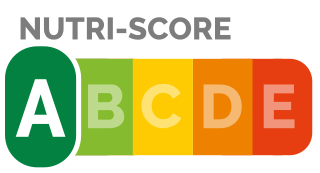# The CAT Scan

The CAT Scan (Cellular Access Test Scan, meow!) is a tool to audit your phone, to simply evaluate which applications ask for too much permissions.

This CAT Scan uses in the database of the open source project Exodus Privacy. This database shows on Android apps the built-in trackers and the amount and nature of permissions needed. More details on the εxodus page.

## But what does CAT Scan do?

The goal of CAT Scan is to make all information more friendly. In other words: to know at a glimpse which applications require too much permissions and are likely to spy on you.

Like any simplification process, CAT has its pros and cons:

• Its main strength is that it is simple and easy to read. From a single glance, you will be able to get an idea of whether an application is intrusive or not, based on the nutriscore, with it colored indications.• Its main weakness is that it is sometimes simplistic. In fact, at times two applications can have a similar score, while one is intrusive and the other requires a lot of permissions, but justifiably so (like Signal, for example).

## Calculation formula

The CAT scan score is based on the following variables

• number of trackers found
• number of “normal” permits
• number of “dangerous” permissions (according to Google’s definition)
• total number of permissions

For each application, a score is calculated according to the following formula.

``[total permissions] + ( [trackers] x [dangerous permissions] )``

The result is ranked on the following scale:

``````A - 0-15
B - 16-30
C - 31-50
D - 51-70
E - 70+``````

To obtain the overall score, the following formula is applied:

``SUM(scores_apps) / [number of applications]``

## And why not another formula?

The idea behind this calculation formula was to base it on the total number of authorizations, and then weigh the score according to the configurations that we consider “dangerous”, i.e. to manage to point out the applications where there are both trackers (which reveal a very likely intrusive approach by the publisher) and critical authorizations (which allow manipulation of sensitive data, such as your address book or your geolocation).

However, as we have explained above, each formula has its pros and cons:

• The strength of this formula is that it is simple and classifies applications effectively (after several trials with other formulas and seeing the results of applications with known privacy practices).
• The weakness of this formula is double.
• This score gives too low a score to some applications that are a priori trustworthy, but which need a large amount of permissions because they have a wide range of functions. This is the case, for example, for some secure messaging applications such as Signal or Element, or, of course, for our own Gao&Blaze game. However, these applications are still few in number, as a significant part of today’s applications are “mono-tasks” and have only one (or a few) main functions.
• It inherits the lacks of Exodus Privacy, i.e., we cannot be 100% sure that all trackers have actually been detected.